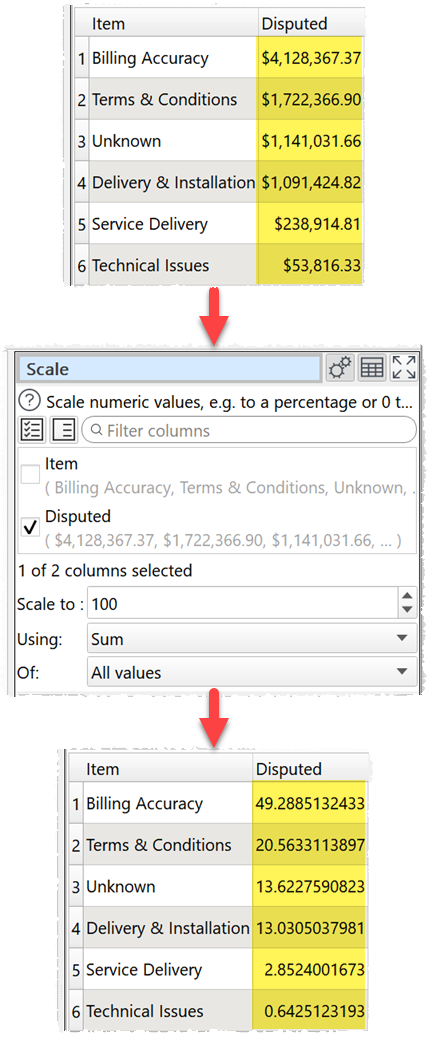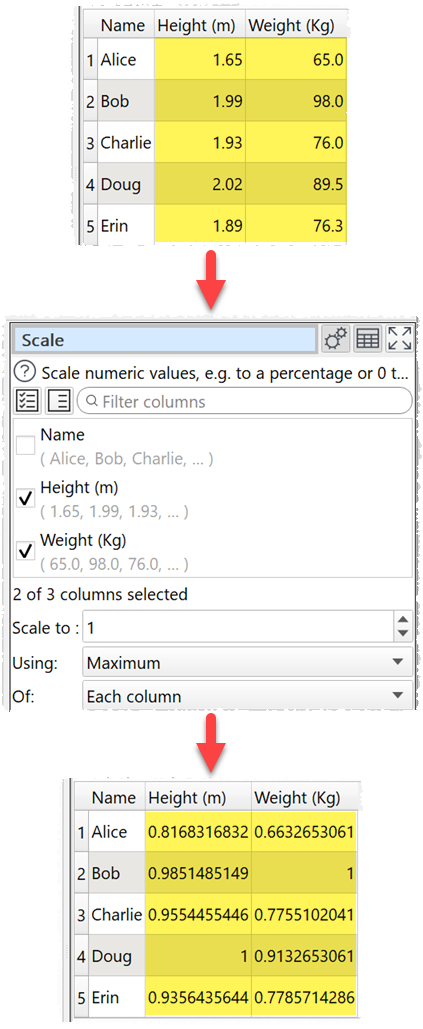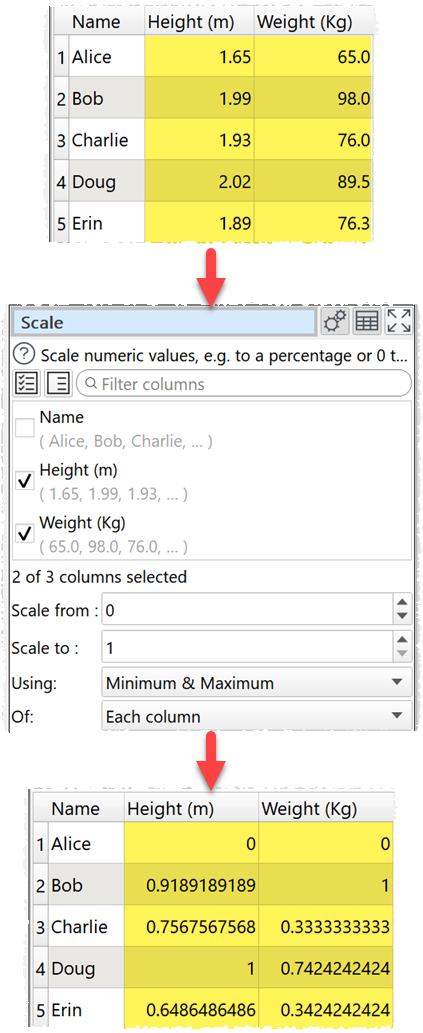﻿ Reference > Transforms > Scale

# Scale

Navigation:  Reference > Transforms >

# Scale

## Description

Scale (normalize) numeric values, e.g. to a percentage or 0 to 1.

## Examples

Scale a single column to a percentage:Scale a table with row, column and grand totals to a percentage (scale to 400 as each value is also added to the row total, column total and grand total):Scale heights and weights so that the largest in each column is 1 and the smallest is 0.Scale heights and weights so that the largest in each column is 1 and the smallest is 0.One.

## Options

Set Columns to the columns whose values you want to scale.

set Scale from to the value you want to scale the minimum value to. E.g. 0 if you want the minimum value scaled to 0. This is only available when Using is set to Minimum and Maximum.

Set Scale to to the value you want to scale the maximum or sum value to. E.g. 100 for a percentage.

Set Using depending on whether you want to use the Sum, Maximum or Minimum and Maximum values for scaling.

Set Of depending on whether you want to scale for Each column, Each row or for All values.

## Notes

Whether values are interpreted as numbers depends on locale.

Non-numeric values (including empty values) are ignored.

To replace empty values with zeros use the Replace transform.

To modify the numerical precision of the results use the Num Format transform.

To add a '%' at the end of values use the Insert transform.

To copy columns before using Scale use Copy Cols .

Warnings are shown in the Warnings tab for non-numeric values.